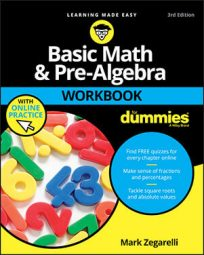##### Basic Math & Pre-Algebra Workbook For Dummies with Online PracticeTo find the volume of a prism or cylinder, you can use the following formula, where Ab is the area of the base and h is the height:
`V = Ab x h`

## Practice questions

1. Find the volume of a prism with a base that has an area of 6 square centimeters and a height of 3 centimeters.
2. Figure out the approximate volume of a cylinder whose base has a radius of 7 millimeters and whose height is 16 millimeters.

1. 18 cubic centimeters
`V = Ab x h = 6cm2 x 3cm = 18cm3`
2. Approximately 2,461.76 cubic millimeters

First, use the area formula for a circle to find the area of the base:

```Ab = π x r2
≅ 3.14 x (7mm)2
= 3.14 x 49mm2
= 153.86mm2```

Plug this result into the formula for the volume of a cylinder:

```V = Ab x h
= 153.86mm2 x 16mm
= 2,461.76mm3```